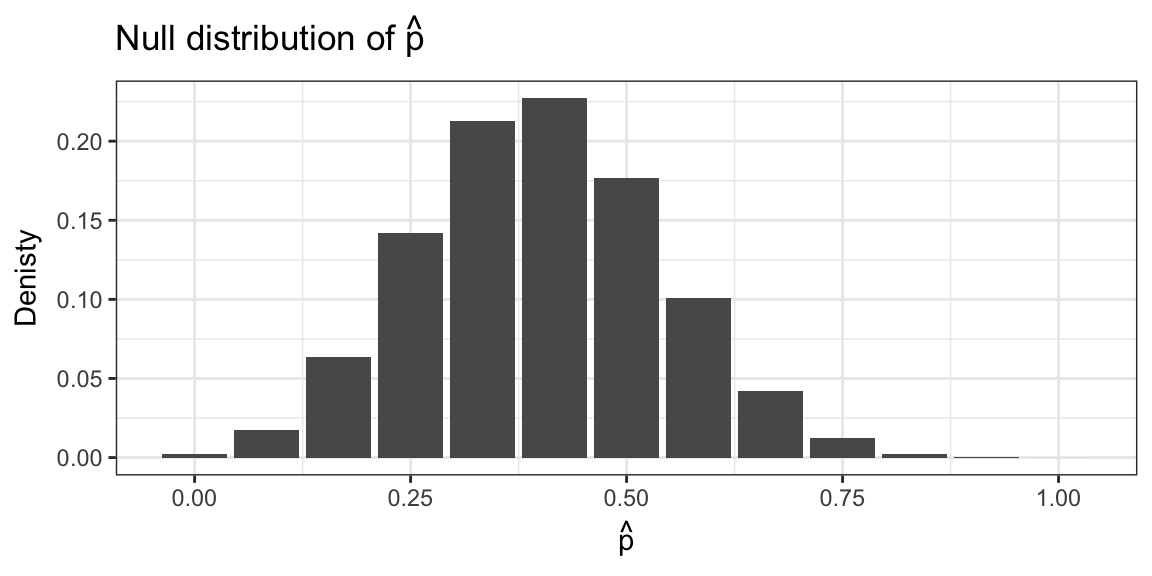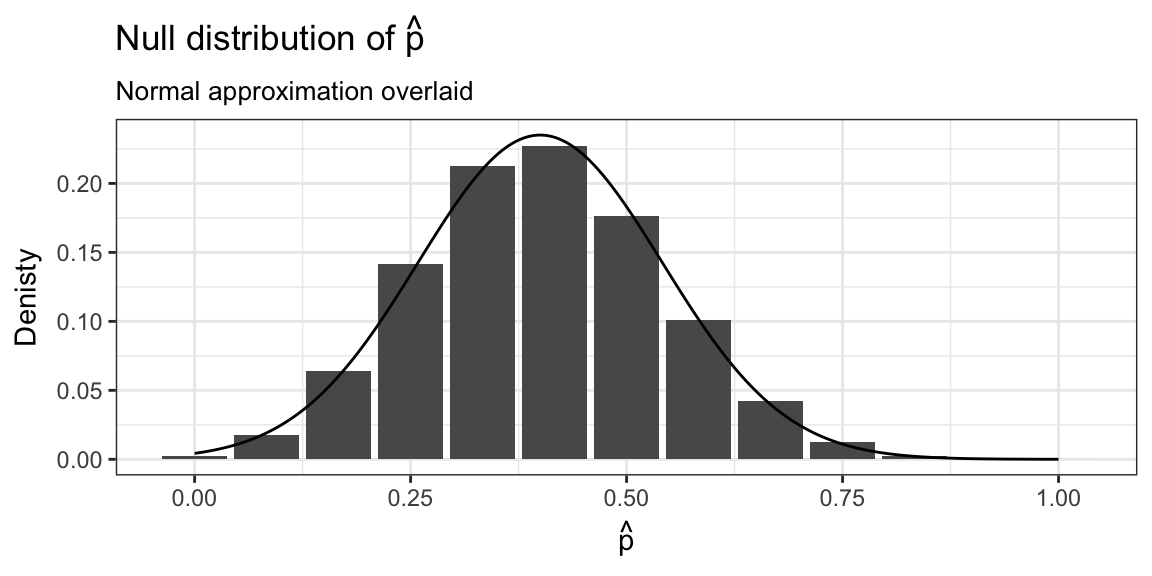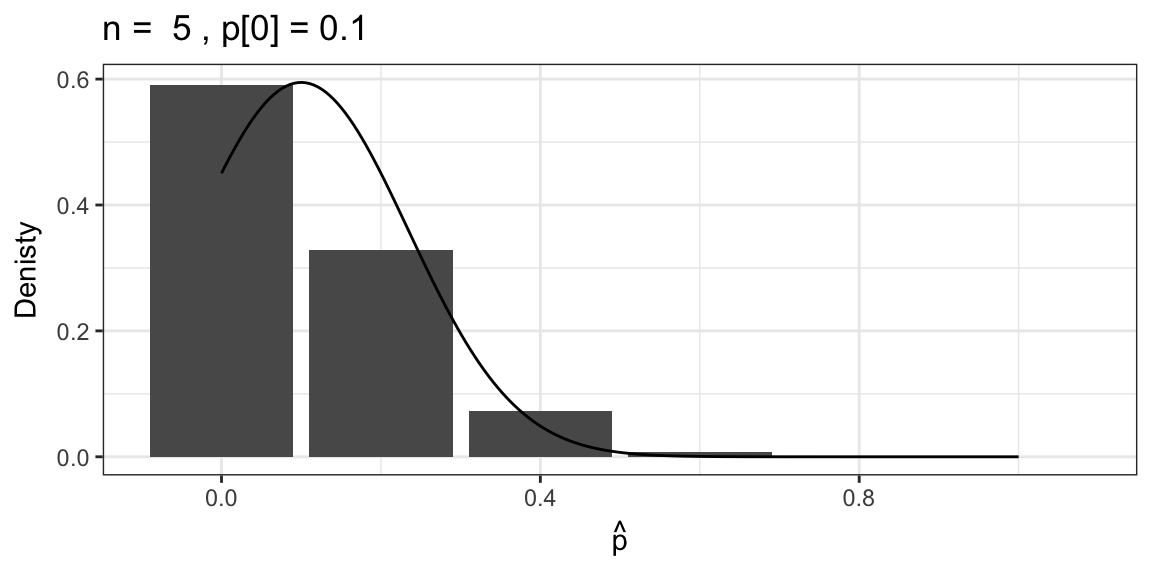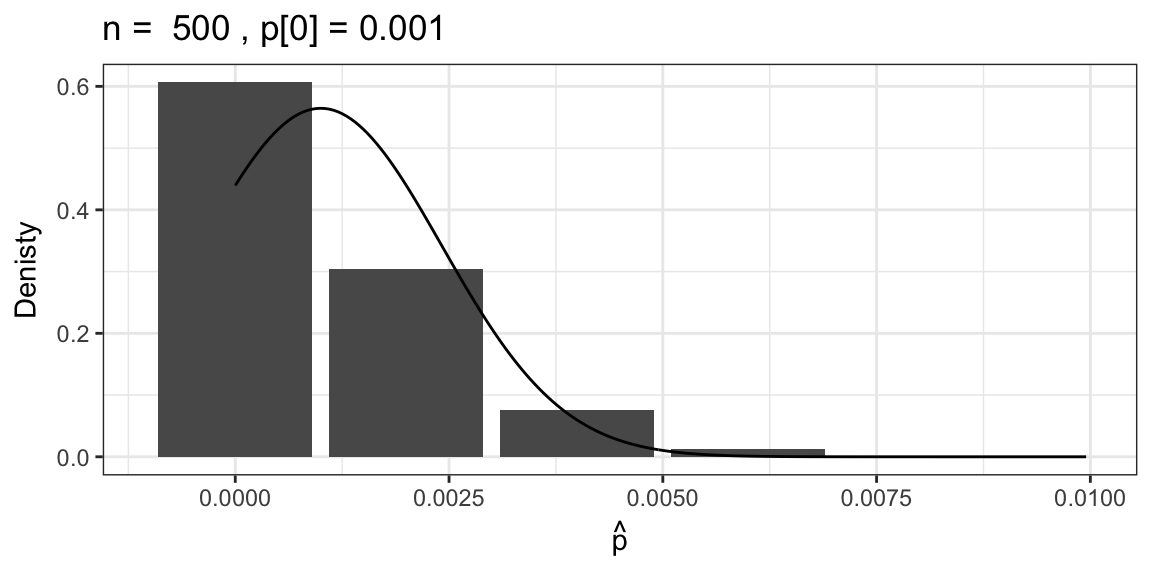# Approximate Binomial Test ST551 Lecture 12

## Midterm

Next Friday Oct 27th in class.

No outside materials except one double-sided page of your own notes and a calculator.

I’ll be putting up a study guide and practice midterm by Friday.

Two options to vote on:

1. No homework due next Thursday
2. No homework due the week after the midterm - won by popular vote

# Finish the CI for last week’s worksheet

## Exact Binomial Test: Takeaways

“Exact” because it uses the exact sampling distribution of the sum of $$Y_i$$.

The actual Type I error rate will never be more than $$\alpha$$, but may be substantially less (i.e. conservative).

You can invert the test to get a confidence interval, but there isn’t an easy closed form for the interval.

## Exact Binomial Test: In R

binom.test(x = 7, n = 12, p = 0.4)
##
##  Exact binomial test
##
## data:  7 and 12
## number of successes = 7, number of trials = 12, p-value = 0.2417
## alternative hypothesis: true probability of success is not equal to 0.4
## 95 percent confidence interval:
##  0.2766697 0.8483478
## sample estimates:
## probability of success
##              0.5833333

## Exact Binomial Test: In R

binom.test(x = 7, n = 12, p = 0.4)

x - count of 1’s, i.e. $$\sum_{i=1}^{n}Y_i$$
n - sample size
p - $$p_0$$, the hypothesized population proportion

The reported CI is a Clopper-Pearson confidence interval, based on the exact distribution but with equal tails (i.e. try to get $$\alpha/2$$ in each tail).

# Approximate Binomial Test

## Approximate Binomial Test

Use fact that: $\overline{Y} \dot\sim N\left( E(Y) , \frac{Var(Y)}{n}\right) = N\left( p , \frac{p(1-p)}{n}\right)$

$Z(p_0) = \frac{\hat{p} - p_0}{\sqrt{p_0 (1 - p_0)/n}}$ $$\hat{p} = \overline{Y}$$ = sample proportion

## Exact distribution of sample proportion## Approximate distribution of sample proportionlibrary(openintro)
census %>%
group_by(sex) %>%
summarise(n = n())
## # A tibble: 2 x 2
##      sex     n
##   <fctr> <int>
## 1 Female   232
## 2   Male   268

Find:

1. $$\hat{p}$$

2. The Z-statistic, for the test of $$H_0: p = 0.5$$

## A confidence interval?

Need to invert test, i.e. find all $$p_0$$ such that: $|Z(p_0)| = \left| \frac{\hat{p} - p_0}{\sqrt{p_0 (1 - p_0)/n}}\right| > z_{1-\alpha/2}$

It’s hard…

$\hat{p} \pm z_{1-\alpha_2} \sqrt{\frac{\hat{p}(1-\hat{p})}{n}}$ Based on inverting a (Wald) test with statistic:

$Z_w(p_0) = \frac{\hat{p} - p_0}{\sqrt{\hat{p} (1 - \hat{p})/n}}$

Asymptotically equivalent to $$Z(p_0)$$ (happens to be the Score test)

library(openintro)
census %>%
group_by(sex) %>%
summarise(n = n())
## # A tibble: 2 x 2
##      sex     n
##   <fctr> <int>
## 1 Female   232
## 2   Male   268

Find:

1. 95% CI for $$p$$.

A score test, $$Z(p_0)$$, might not agree with a Wald interval.

Learn to live with it…or don’t calculate things by hand.

## In R

prop.test(x = 232, n = 232 + 268, p = 0.5, correct = FALSE)
##
##  1-sample proportions test without continuity correction
##
## data:  232 out of 232 + 268, null probability 0.5
## X-squared = 2.592, df = 1, p-value = 0.1074
## alternative hypothesis: true p is not equal to 0.5
## 95 percent confidence interval:
##  0.4207282 0.5078208
## sample estimates:
##     p
## 0.464

## In R

prop.test(x = 232, n = 232 + 268, p = 0.5, correct = FALSE)

Equivalent to $$Z(p_0)$$ and inverts to get confidence interval (i.e. p-value and CI will agree).

Reports X-squared, $$\chi^2$$ statistic, take square root to get $$Z$$

## When to use the Approximate Binomial test?

Compare to:

binom.test(x = 232, n = 232 + 268, p = 0.5)
##
##  Exact binomial test
##
## data:  232 and 232 + 268
## number of successes = 232, number of trials = 500, p-value =
## 0.1174
## alternative hypothesis: true probability of success is not equal to 0.5
## 95 percent confidence interval:
##  0.4196128 0.5088153
## sample estimates:
## probability of success
##                  0.464

## When to use the Approximate Binomial test?The approximation isn’t great for small expected counts.

OK to use the approximation if: $$np_0 > 5$$ and $$n(1-p_0)> 5$$

(Or something similar)

## Next time…

Use Binomial test as a way to look at population median.